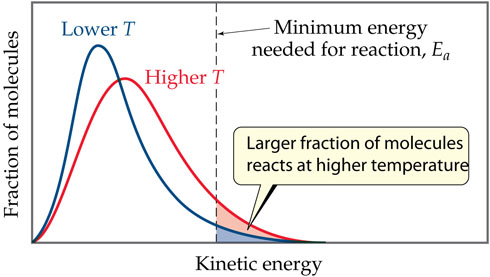# Problem:  The graph shown displays the effect of two different temperatures on the distribution of kinetic energies of molecules in a sample. One temperature is named "Lower T" and is graphed in blue. The other temperature is named "Higher T" and is graphed in red.If there was a third, new temperature that would be graphed in green and would display the effect of a greater, even higher temperature than "Higher T" on the distribution of kinetic energies of molecules in a sample, describe the shape and relative height of this new green curve as it relates to the existing red "Higher T" curve in the graph.

###### FREE Expert Solution

We’re being asked which gas has the largest molar mass at 25 ˚C.

In the graph, the y-axis is the fraction of molecules while the x-axis is the kinetic energy. According to the kinetic molecular theory of gases, the average kinetic energy of a gas is directly proportional to the temperature of the gas: a higher temperature results in a higher average kinetic energy.

Checking the graph:###### Problem DetailsThe graph shown displays the effect of two different temperatures on the distribution of kinetic energies of molecules in a sample. One temperature is named "Lower T" and is graphed in blue. The other temperature is named "Higher T" and is graphed in red.

If there was a third, new temperature that would be graphed in green and would display the effect of a greater, even higher temperature than "Higher T" on the distribution of kinetic energies of molecules in a sample, describe the shape and relative height of this new green curve as it relates to the existing red "Higher T" curve in the graph.